## GOING IN CIRCLES [DAY 6]

### EXPLORING PI: CIRCUMFERENCE & AREA OF CIRCLES

Leverage contextual problems to investigate and emerge Pi to help conceptualize formulas for both the circumference and are of a circle.

## Intentionality & Unit Overview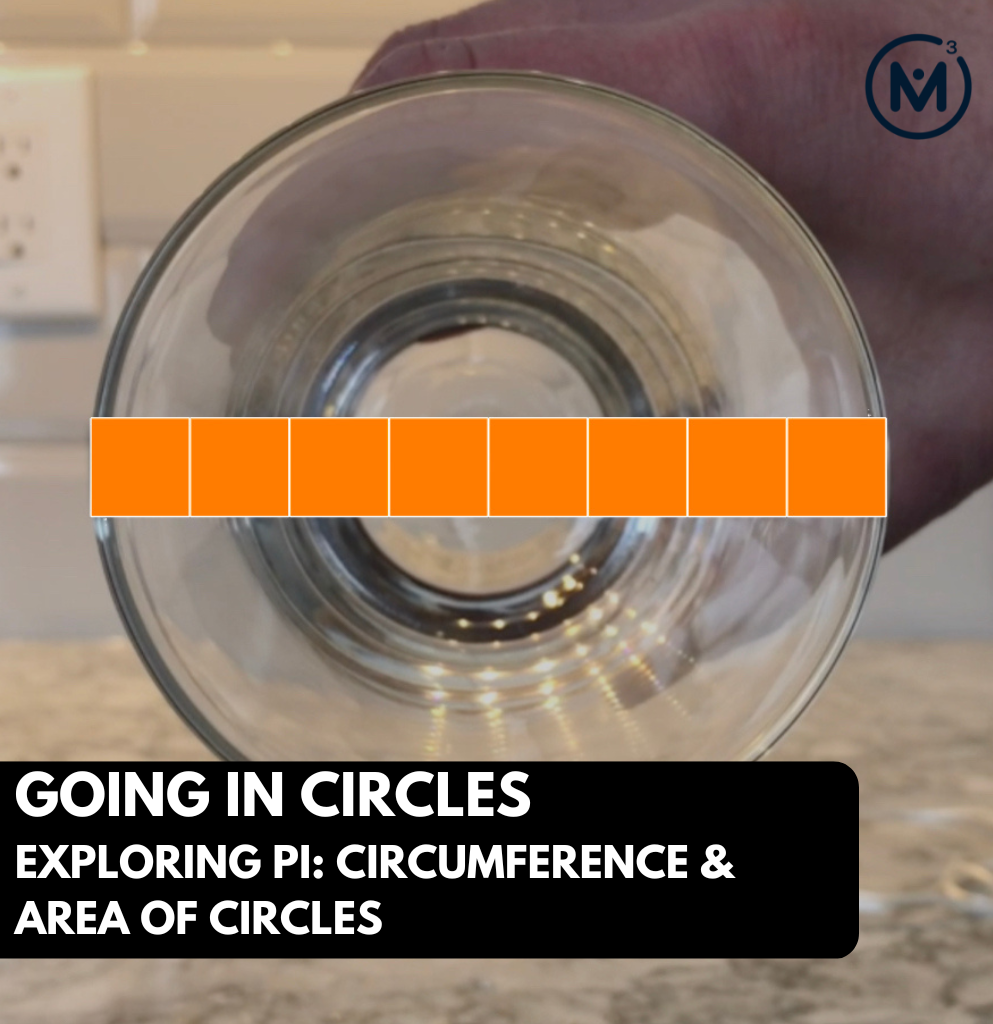### Access each lesson from this unit using the navigation links below

Students will deepen their understanding and develop their skills related to circumference, diameter and area of a circle.

## Intentionality…

Through this purposeful practice, students will apply the concept of circumference and area of a circle to deepen understanding and begin building procedural fluency related to big ideas including the following:

• When exploring measurement relationships of any circle, there are three (3) standard measures that can be used to reveal more information about the circle:
• Circumference: the perimeter of a circle.
• Diameter: any straight line segment beginning and ending on the outer edge of a circle and passes through its centre.
• Radius: any straight line segment beginning at the centre of a circle and ending on the outer edge of the circle.
• Knowing one of the standard measures of a circle provides information about all other measures;
• There is a multiplicative relationship between the length of the diameter and the circumference of a circle. In other words, as the diameter of a circle increases, the circumference increases multiplicatively (not additively);
• For all circles, the ratio of the circumference to the diameter is the same (approximately 22:7); and,
• For all circles, the rate of each unit of circumference length per unit of diameter length is the same (approximately $$\frac{22}{7}$$or 3.14) and is known as Pi, π.
• The area of a circle can be determined by decomposing and recomposing the figure into familiar polygons (i.e.: rectangles or parallelograms); and,
• For all circles, changing the diameter or radius will affect the area and circumference in predictable ways.

## String of Related Visual Number Talk Prompts

In the number talk for today, students will be given an opportunity to build their flexibility and fluency with circle measurement. In particular, students will be building on their understanding of the ratio between circumference and the diameter and revisit how we can use these measures to approximate the area of a circle.
Early in the string of related visual number talk prompts, we have students approximate the area using their spatial reasoning first, then confirm through the decomposition of the circle into a parallelogram or rectangle. As students move through the prompts, we then encourage students to improve their precision through the use of fluency and flexibility with the dimensions of a circle and the corresponding dimensions of a decomposed/recomposed rectangle.
It can be helpful to craft a context that students can leverage throughout this string. For example, using the Tile Circle context from Days 2 and 5 or the Pizza conext from Day 4 can be helpful.

You might consider facilitating this number talk orally or consider leveraging the visual number talk prompts included to assist in your facilitation.

Here is the string of number talk prompts:

What is the approximate area of a circle with a diameter of 4 units? Make an estimate…

What is the approximate area of a circle with a radius of 4 units? Make an estimate…

What is the approximate area of a circle with a diameter of 10 units? Make an estimate…

What is the approximate circumference of a circle with an area of 75 square units? Make an estimate…

What is the approximate area of a circle when the area of the radius-squared is 36 square units? Make an estimate…

Students are welcome to use any approach of their choice, but they must still justify their thinking by modelling without the use of a calculator.
Some students may be confident using the area of a circle formula, A = $$πr^2$$, however we do want them to explain and model why this formula works.

Regardless of whether you choose to orally facilitate this number talk or leverage the visual number talk prompts, be sure to record student thinking publicly for all to see including the symbolic representation of the work they are engaging in from this string as you go.

Encourage or create opportunities to represent student thinking using the double number line by pausing questions such as “would it be ok if I show your thinking using this area model” if students do not initiate the use of a model. If students want to model their thinking another way however, this should be honoured not discouraged. Through this string, encourage students to recognize that the formula for determining the area of a circle is derived by decomposing the circle into another known simple shape (a rectangle or parallelogram).

## Visual Math Talk Prompt #1

Begin playing the following visual number talk prompt video and be ready to pause the video where indicated to allow for think time.

Share the following prompt both visually on the screen and verbally:

What is the approximate area of a circle with a diameter of 4 units?
Make an estimate.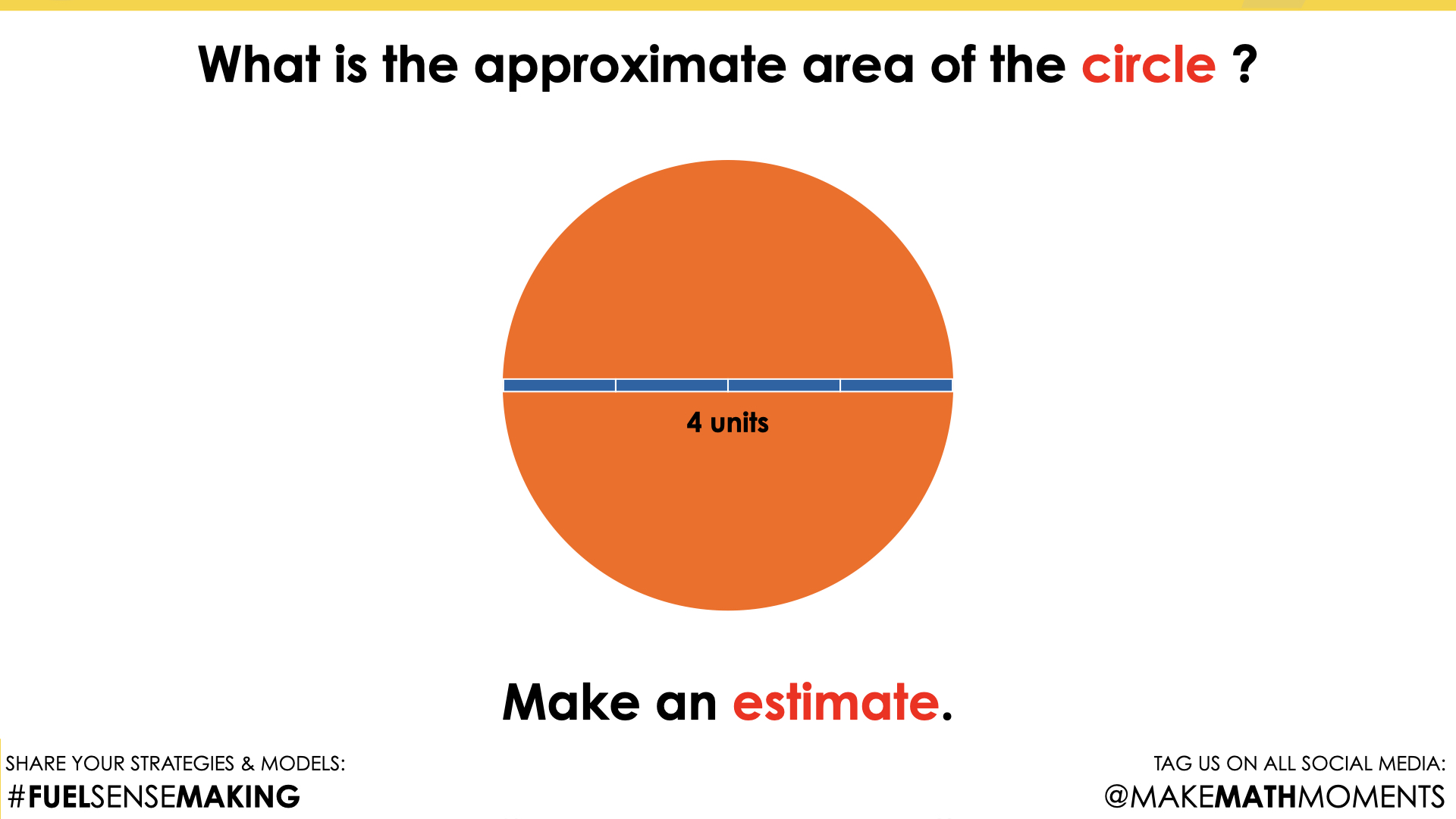By this point in the unit, the hope is that all students have build fluency and flexibility with the relationship between the length of the circumference and the length of the diameter of any circle and therefore can quickly approximate the circumference to be “a little more than” 3 times the diameter, or about 13 units long.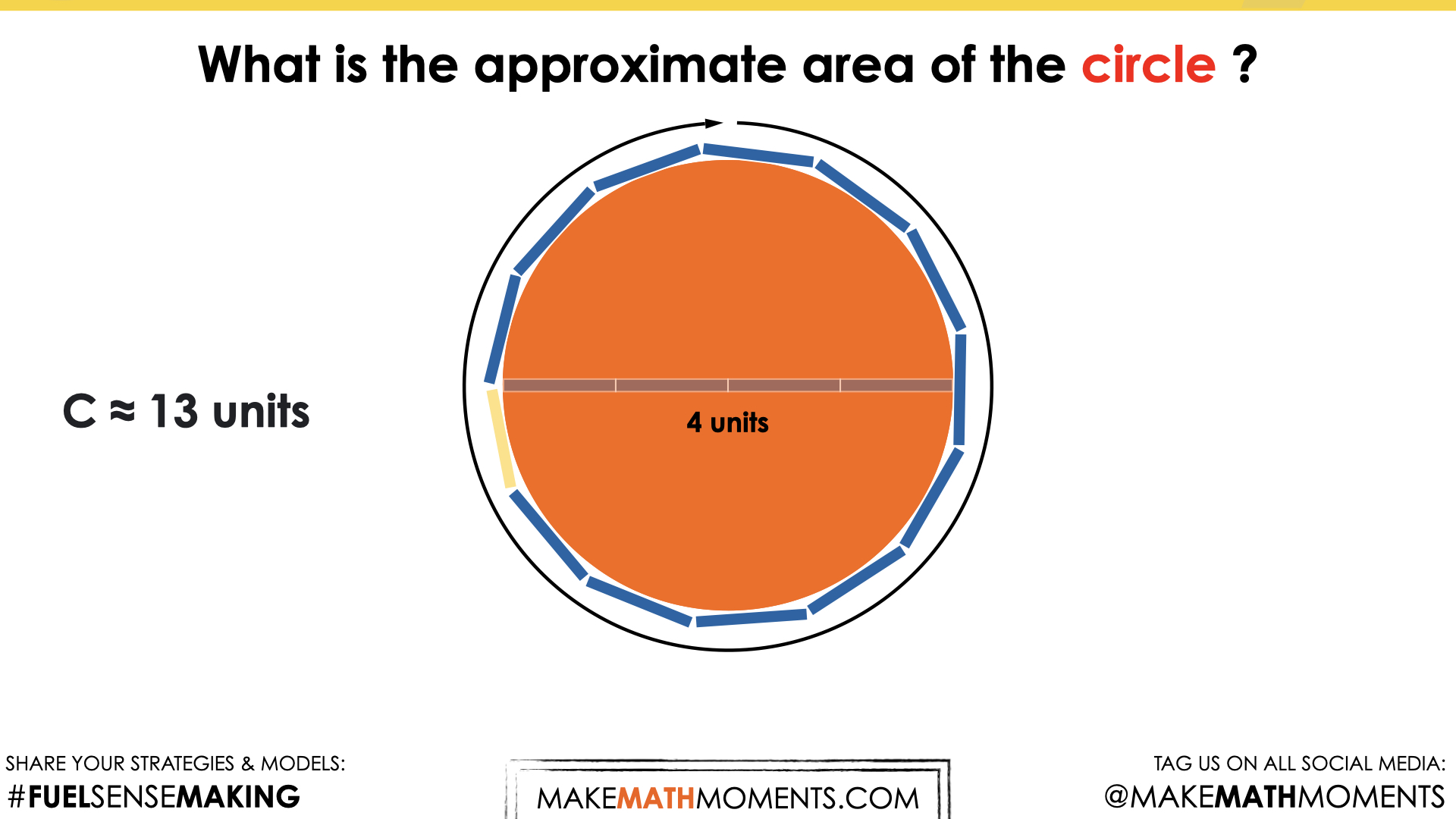From here, it is likely that you will have some students who want to rush to the algorithm and simply plug in 4 units and π (or about 3.14) into the formula, A = $$πr^2$$, which is fine. However, encourage them to be convincing which will require them to model how the formula is derived by decomposing and recomposing the circle into a more friendly shape.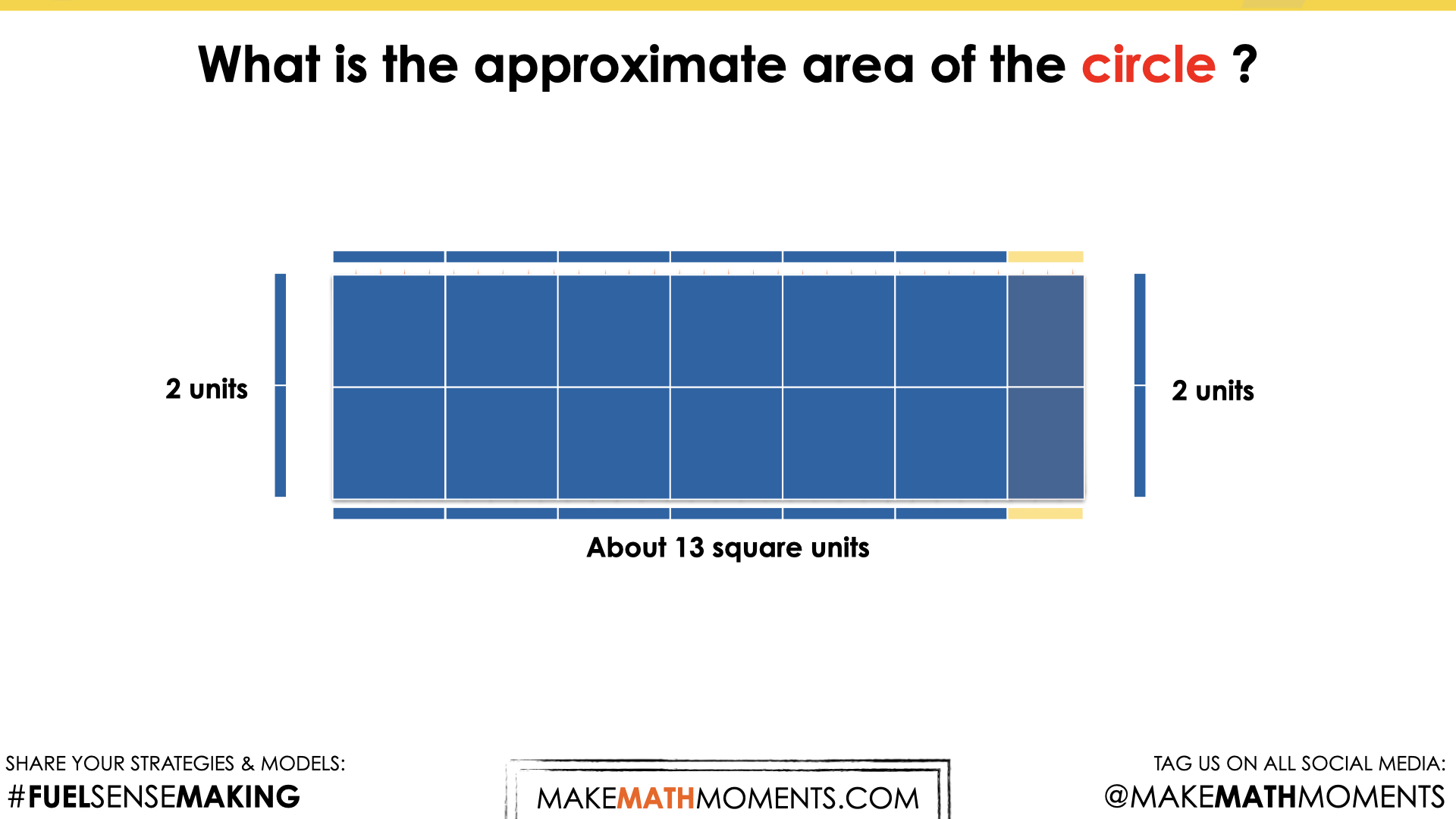For others, they might still be at a place where they are spatially visualizing how many of the square units will cover the circle (or rectangle). Encouraging students to articulate how they are iterating the square units over the circle (or rectangle) is helpful to ensure that all students are connecting what finding the area truly represents and how it connects to the more abstract representation (i.e.: the formula).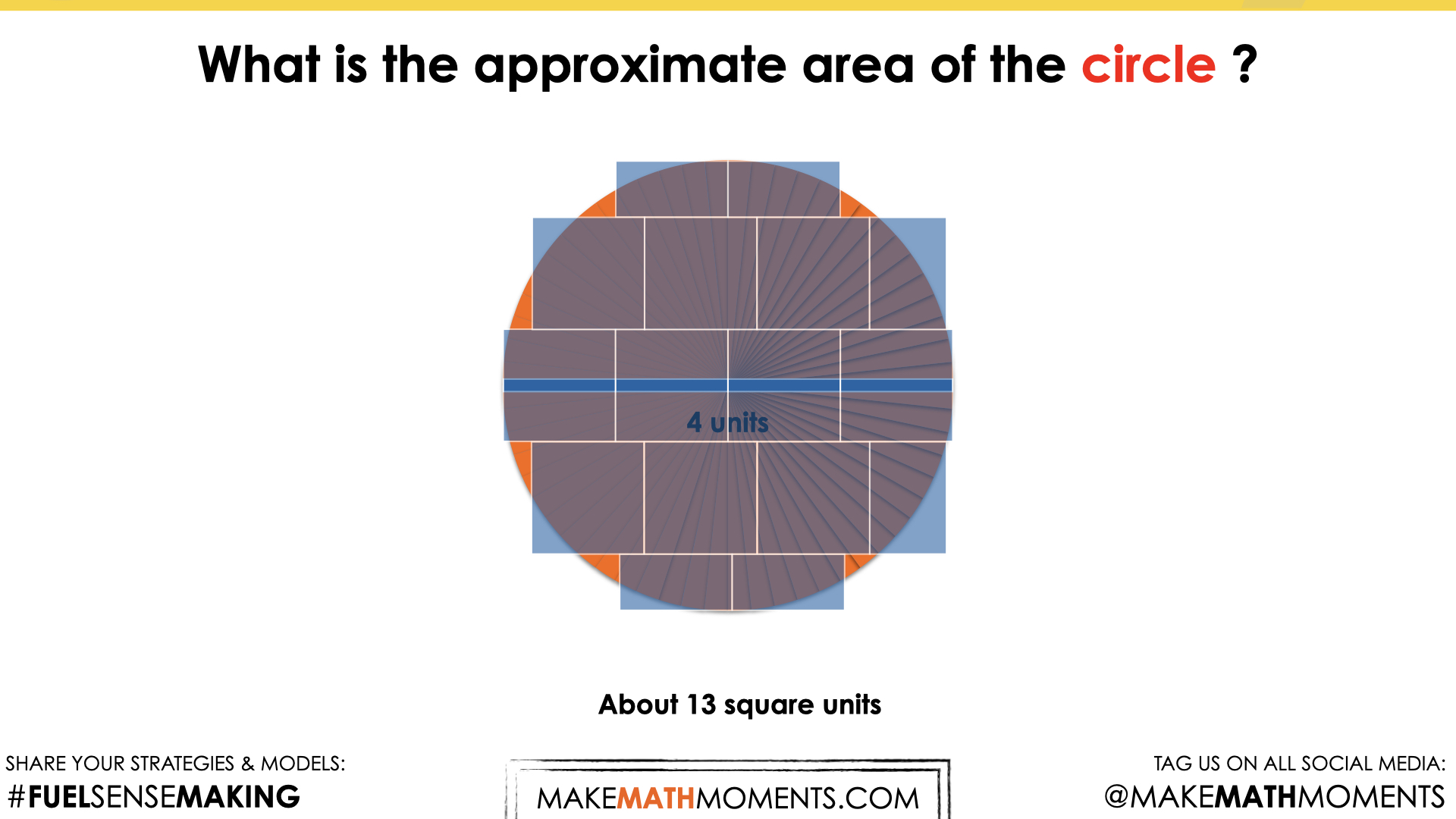Whether students are spatially iterating the unit square over the circle, over the circle recomposed as a rectangle, or whether they are already using the formula, students should come up with a reasonable approximation of about 12 to 13 square units.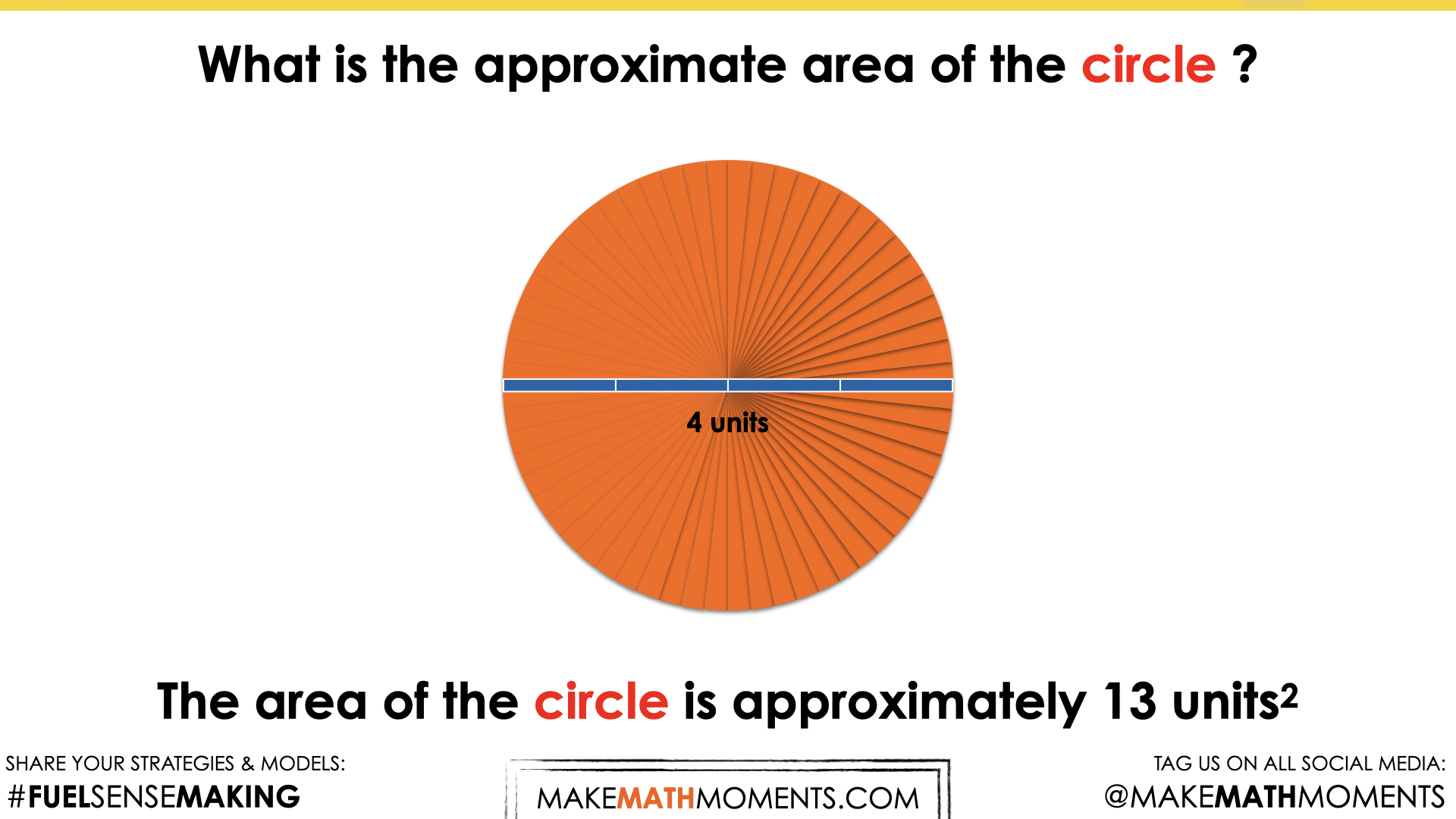## Visual Math Talk Prompt #2

Move on to the next visual number talk prompt video.

Share the following prompt both visually on the screen and verbally:

What is the approximate area of a circle with a radius of 4 units?
Make an estimate.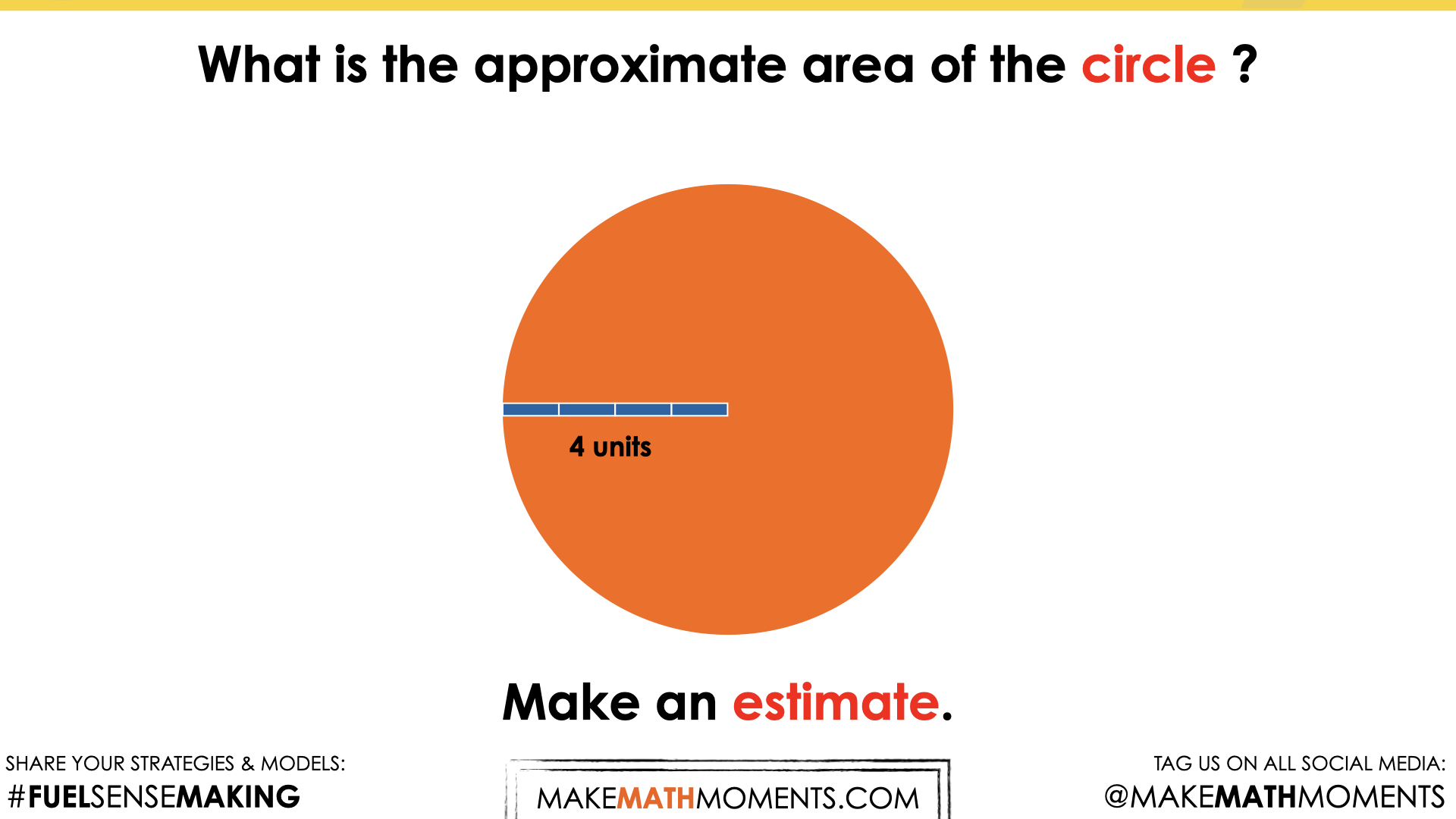Remind students that the goal for this estimate is to get them visualizing what it means to determine the area of the circle. Some students might visualize square units iterated over the circle, however we want them to start envisioning what the decomposed circle will look like when it is recomposed into a rectangle.
Soon enough, students will begin to realize that the circle recomposed as a rectangle will have dimensions of the radius by half of the circumference. Therefore, before we can approximate with more precision than simply visually iterating square units all over the circle or rectangle, we must determine the circumference of the circle by approximately tripling the length of the diameter.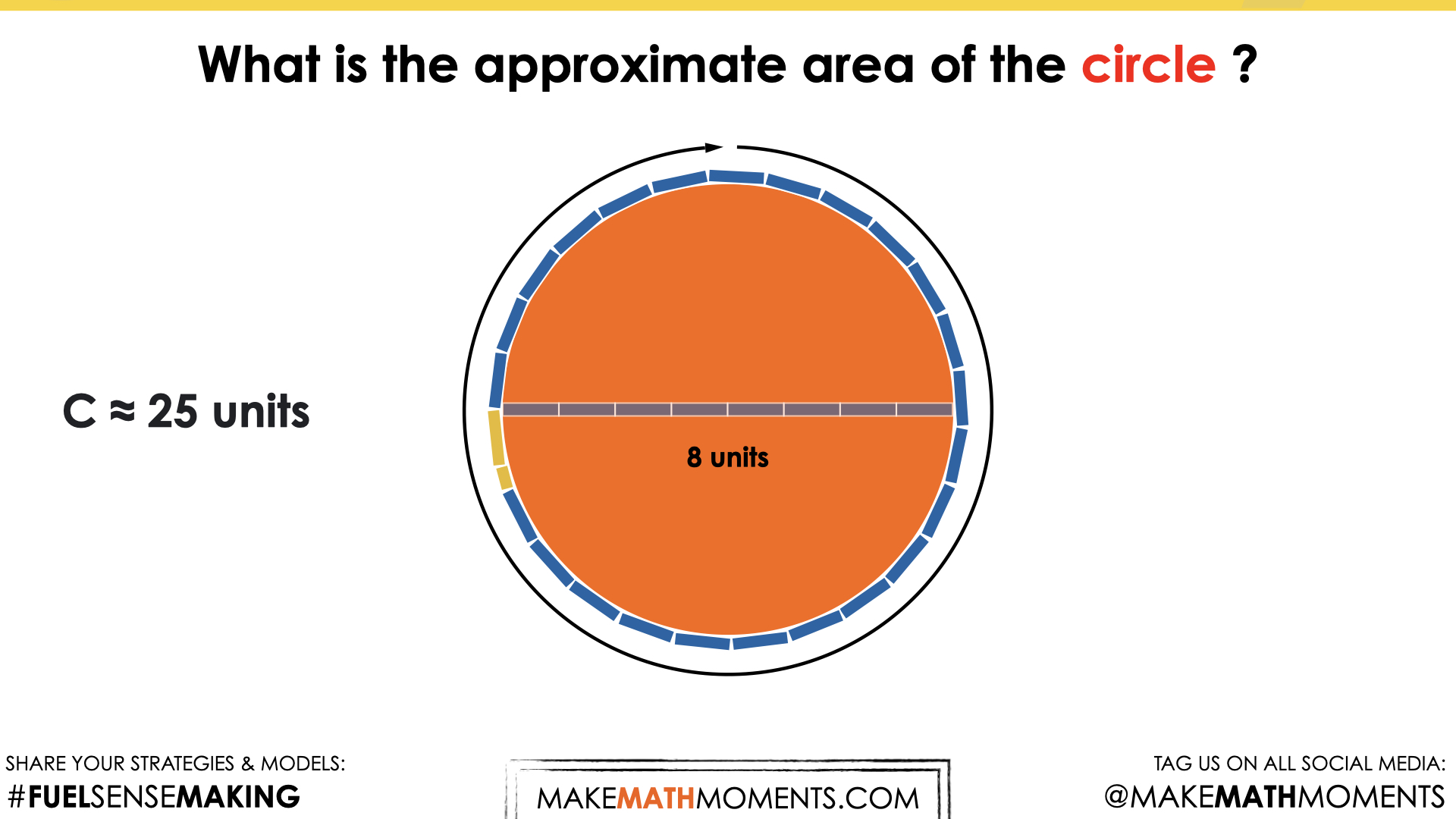As we decompose the circle and recompose it into a rectangle, we end up with the familiar dimensions of the radius by half the circumference which can be easily used to approximate the area.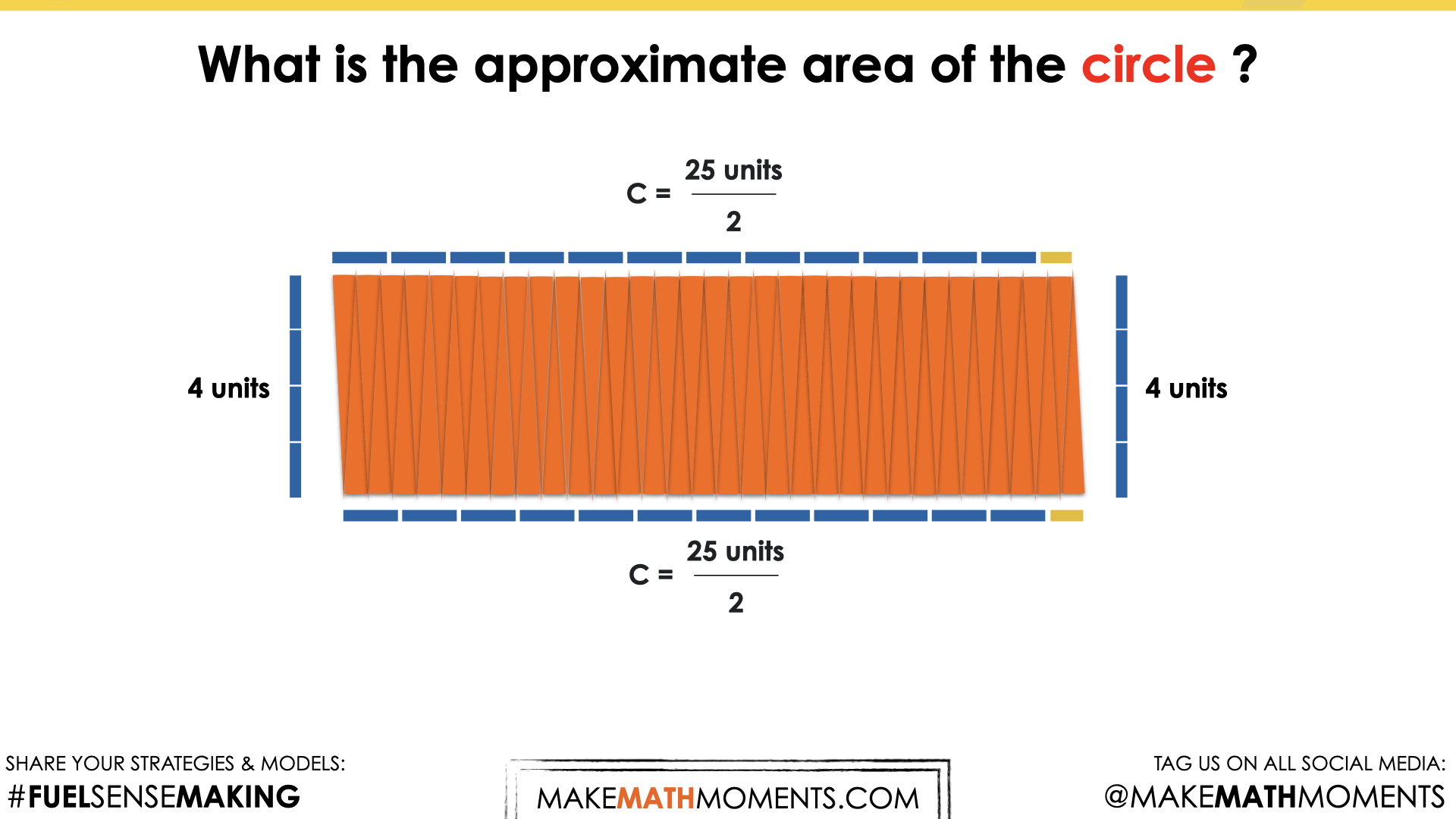As the facilitator, we want to begin asking students to notice how the radius lives within the length of half the circumference. In particular, you might ask students to approximate how many radius lengths are in the length of half the circumference. They should notice that there are a little more than 3 radius lengths. From here, some students might realize that this is equivalent to π. If they do not, be sure to highlight this so students can begin to make a connection to the actual formula we are using to find the area of a circle, $$πr^2$$.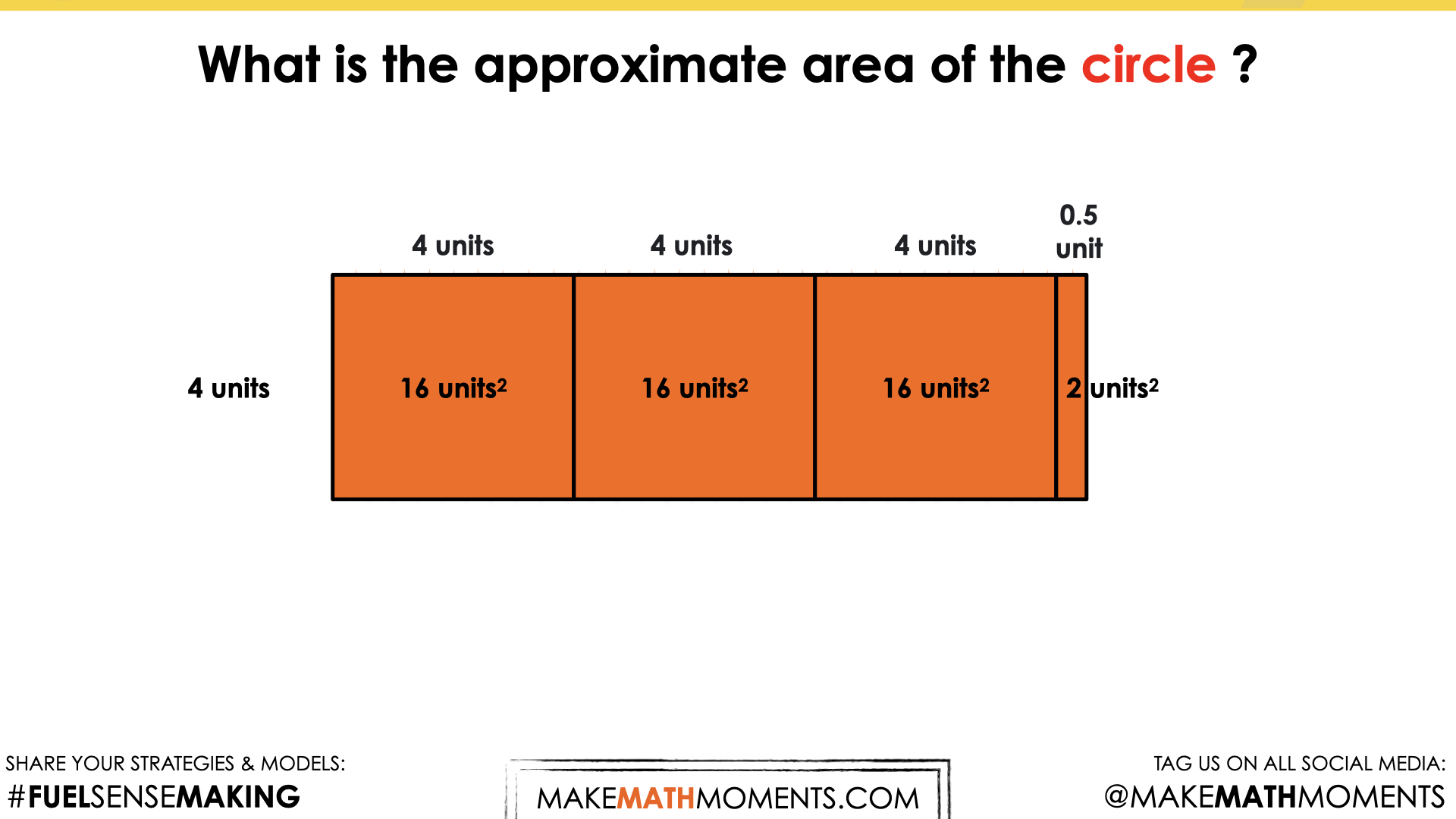This will hopefully help all students begin to conceptually understand a formula for area of a circle – whether they were already using the formula to find area or whether they were still decomposing the circle into a rectangle.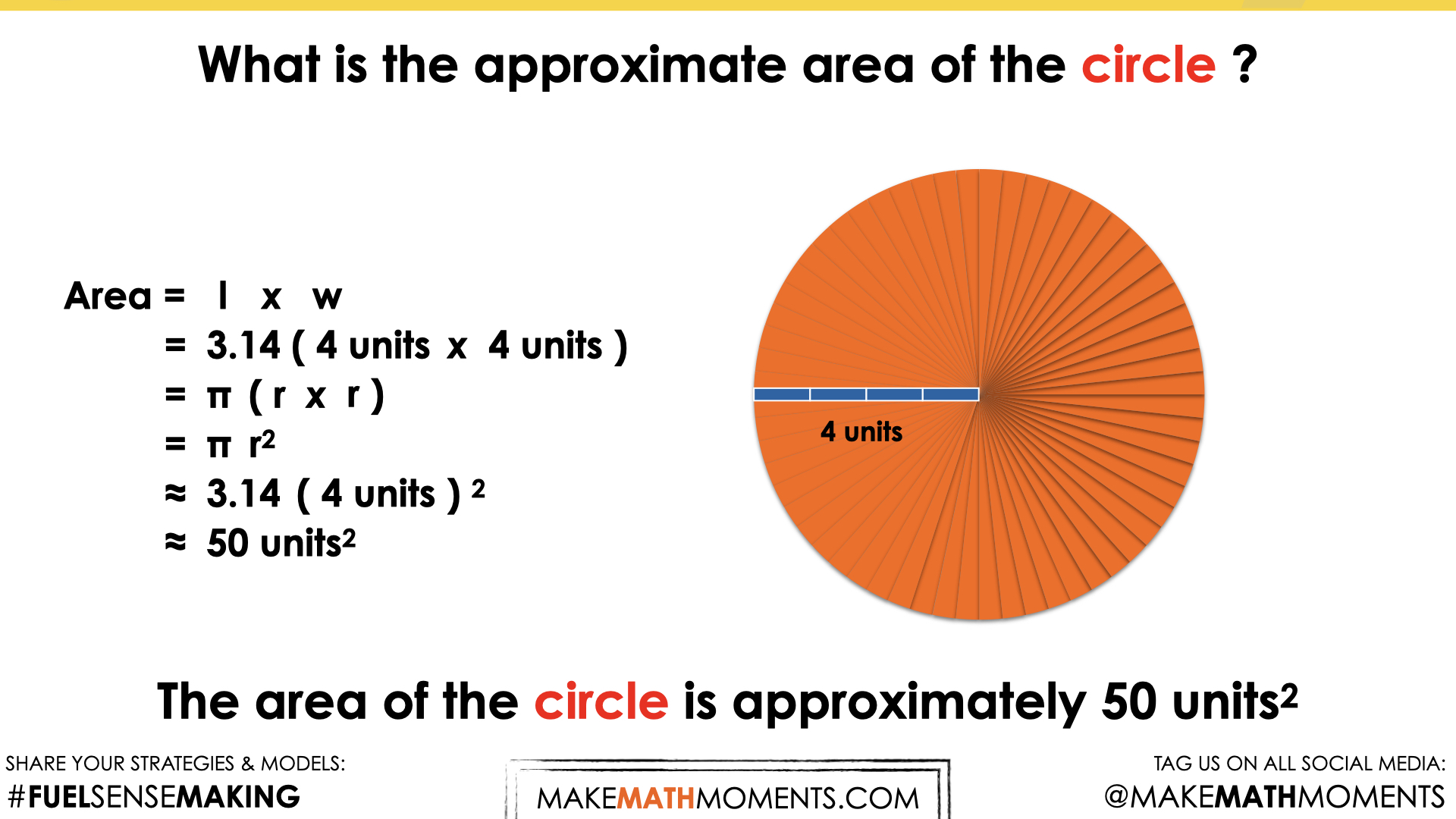## Visual Math Talk Prompt #3

Begin playing the next visual number talk prompt video.

Share the following prompt both visually on the screen and verbally:

What is the approximate area of a circle with a diameter of 10 units?
Make an estimate.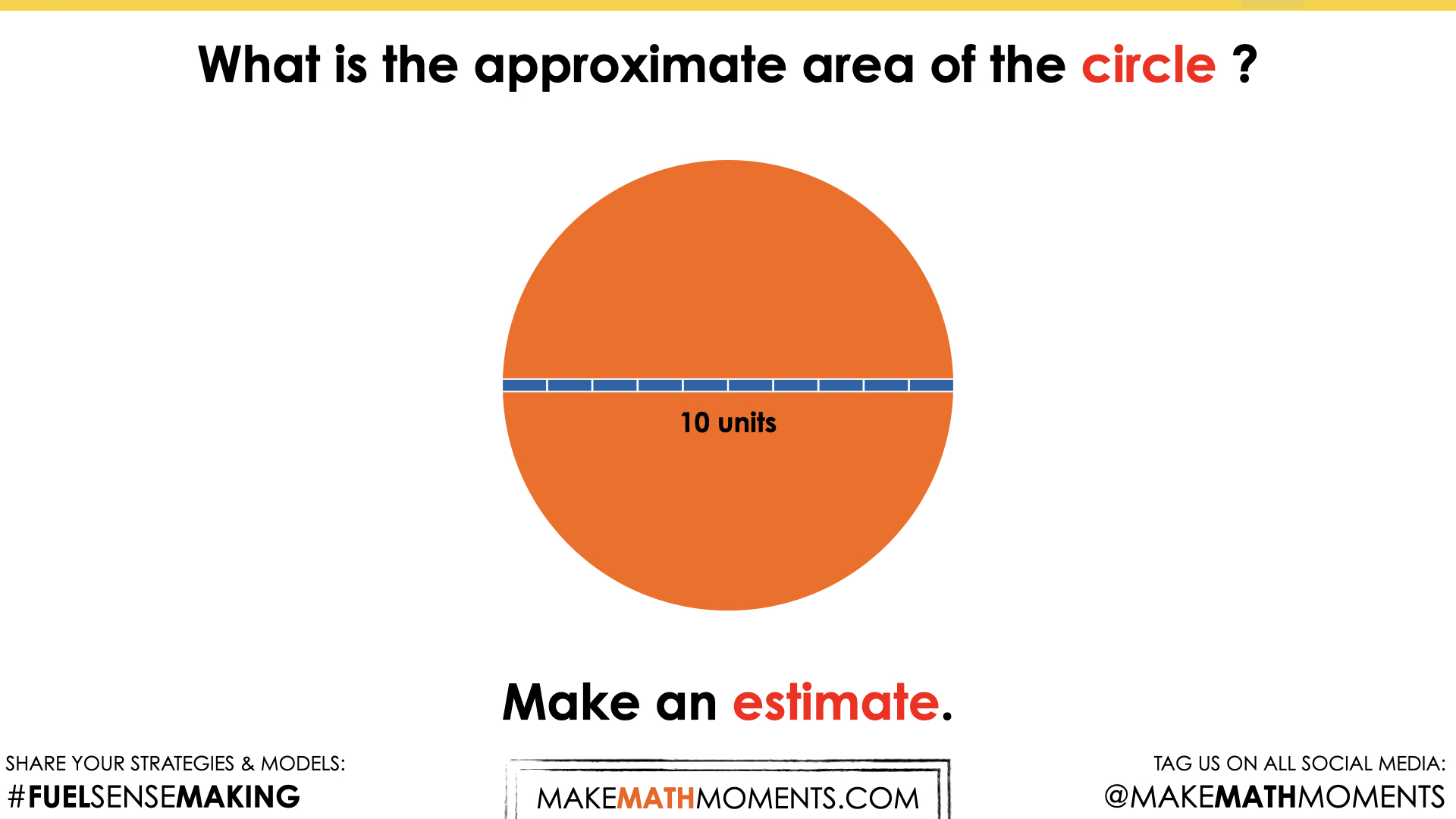Hopefully, students will leverage various approaches by using visual models and/or the formula to help them approximate the area of the circle.

Of course, the facilitator must meet all students where they are and continue to highlight connections to ensure that all students make connections across the visual and symbolic representations. This means that students who are not leveraging the formula, must also be nudged to begin to see the formula coming to life. On the other hand, students leveraging the formula must also be able to verbally (or visually) demonstrate why multiplying the area of the radius-squared by π will result in the area (i.e.: the circle can be recomposed as a rectangle with about 3.14 groups of a square with the dimensions of the radius by the radius).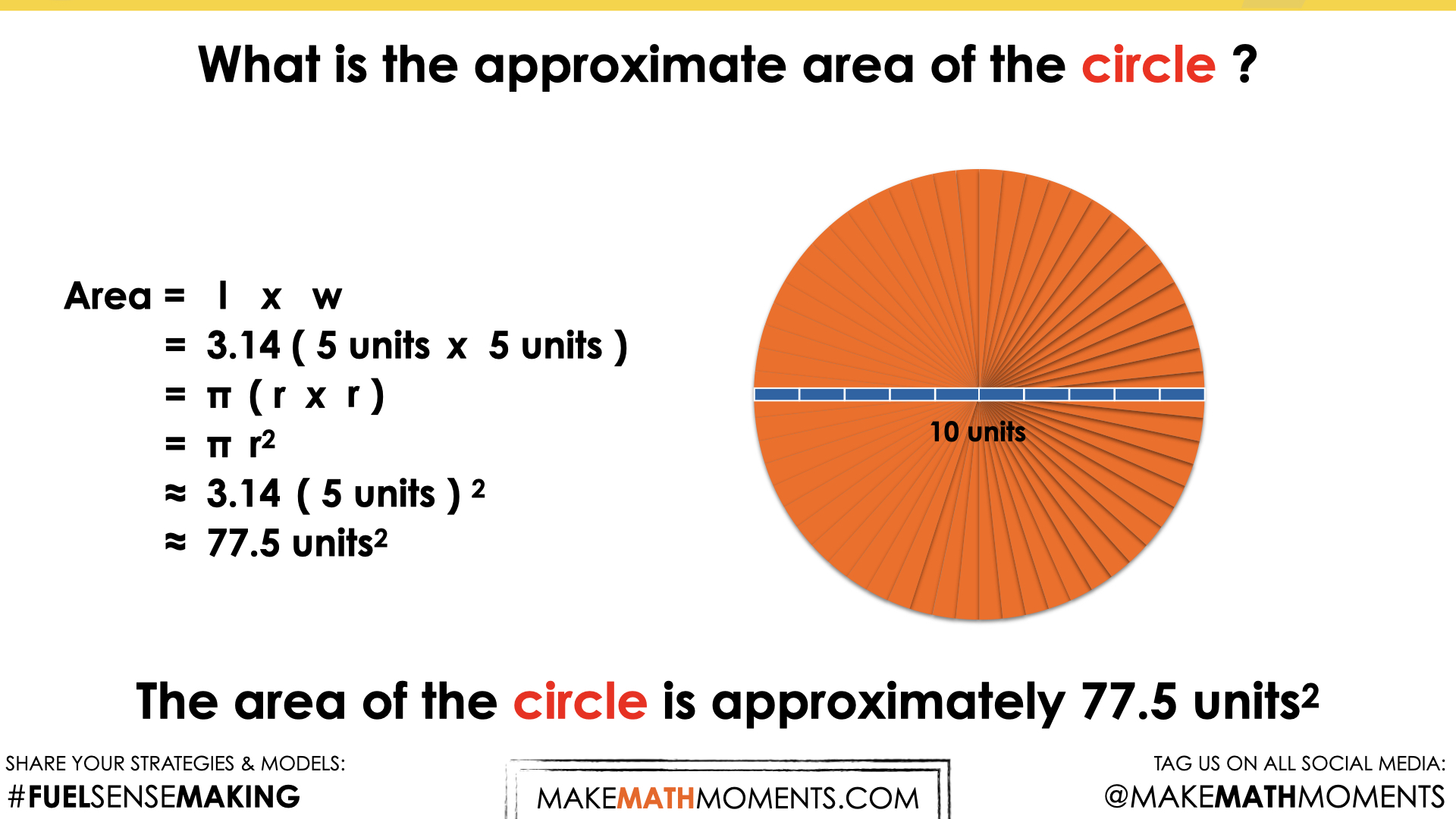## Visual Math Talk Prompt #4

Login/Join to access two (2) additional Visual Math Talk Prompts as well as the Teacher Guide, downloadable slide decks and printable handouts for this lesson and all problem based units.

## Visual Math Talk Prompt #5

Login/Join to access two (2) additional Visual Math Talk Prompts as well as the Teacher Guide, downloadable slide decks and printable handouts for this lesson and all problem based units.

## Working With Circles: Area

Login/Join to access the Teacher Guide, downloadable slide decks and printable handouts for this lesson and all problem based units.

## Practice Problems: Area of a Circle

Login/Join to access the Teacher Guide, downloadable slide decks and printable handouts for this lesson and all problem based units.

Login/Join to access the entire Teacher Guide, downloadable slide decks and printable handouts for this lesson and all problem based units.

## Educator Discussion Area

Login/Join to access the entire Teacher Guide, downloadable slide decks and printable handouts for this lesson and all problem based units.

## Explore Our 60+ Problem Based Units

This Make Math Moments Lesson was designed to spark curiosity for a multi-day unit of study with built in purposeful practice, number talks and extensions to elicit and emerge strategies and mathematical models.

Dig into our other units of study and view by concept continuum, grade or topic!